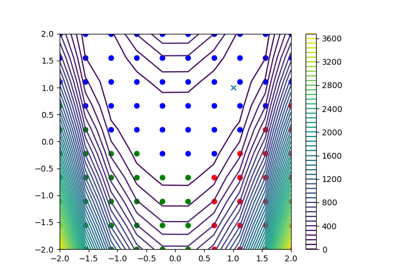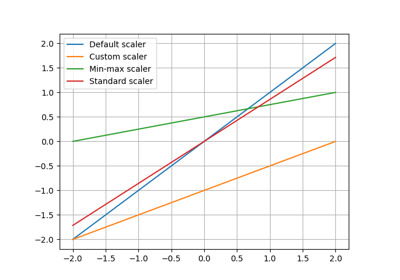gemseo / mlearning / transform / scaler

# scaler module¶

Scaling a variable with a linear transformation.

The Scaler class implements the default scaling method applying to some parameter $$z$$:

$\bar{z} := \text{offset} + \text{coefficient}\times z$

where $$\bar{z}$$ is the scaled version of $$z$$. This scaling method is a linear transformation parameterized by an offset and a coefficient.

In this default scaling method, the offset is equal to 0 and the coefficient is equal to 1. Consequently, the scaling operation is the identity: $$\bar{z}=z$$. This method has to be overloaded.

class gemseo.mlearning.transform.scaler.scaler.Scaler(name='Scaler', offset=0.0, coefficient=1.0)[source]

Bases: Transformer

Data scaler.

Parameters:
• name (str) –

A name for this transformer.

By default it is set to “Scaler”.

• offset (float | ndarray) –

The offset of the linear transformation.

By default it is set to 0.0.

• coefficient (float | ndarray) –

The coefficient of the linear transformation.

By default it is set to 1.0.

compute_jacobian(data, *args, **kwargs)

Force a NumPy array to be 2D and evaluate the function f with it.

Parameters:
• data (ndarray) – A 1D or 2D NumPy array.

• *args (Any) – The description is missing.

• **kwargs (Any) – The description is missing.

Returns:

Any kind of output; if a NumPy array, its dimension is made consistent with the shape of data.

Return type:

Any

compute_jacobian_inverse(data, *args, **kwargs)

Force a NumPy array to be 2D and evaluate the function f with it.

Parameters:
• data (ndarray) – A 1D or 2D NumPy array.

• *args (Any) – The description is missing.

• **kwargs (Any) – The description is missing.

Returns:

Any kind of output; if a NumPy array, its dimension is made consistent with the shape of data.

Return type:

Any

duplicate()

Duplicate the current object.

Returns:

A deepcopy of the current instance.

Return type:

Transformer

fit(data, *args)

Fit the transformer to the data.

Parameters:
• data (ndarray) – The data to be fitted, shaped as (n_observations, n_features) or (n_observations, ).

• args (Union[float, int, str]) –

Return type:

None

fit_transform(data, *args)

Fit the transformer to the data and transform the data.

Parameters:
• data (ndarray) – The data to be transformed, shaped as (n_observations, n_features) or (n_observations, ).

• args (Union[float, int, str]) –

Returns:

The transformed data, shaped as data.

Return type:

ndarray

inverse_transform(data, *args, **kwargs)

Force a NumPy array to be 2D and evaluate the function f with it.

Parameters:
• data (ndarray) – A 1D or 2D NumPy array.

• *args (Any) – The description is missing.

• **kwargs (Any) – The description is missing.

Returns:

Any kind of output; if a NumPy array, its dimension is made consistent with the shape of data.

Return type:

Any

transform(data, *args, **kwargs)

Force a NumPy array to be 2D and evaluate the function f with it.

Parameters:
• data (ndarray) – A 1D or 2D NumPy array.

• *args (Any) – The description is missing.

• **kwargs (Any) – The description is missing.

Returns:

Any kind of output; if a NumPy array, its dimension is made consistent with the shape of data.

Return type:

Any

CROSSED: ClassVar[bool] = False

Whether the fit() method requires two data arrays.

property coefficient: ndarray

The scaling coefficient.

property is_fitted: bool

Whether the transformer has been fitted from some data.

name: str

The name of the transformer.

property offset: ndarray

The scaling offset.

property parameters: dict[str, Union[bool, int, float, numpy.ndarray, str, NoneType]]

The parameters of the transformer.

## Examples using Scaler¶Quality measure for surrogate model comparison

Quality measure for surrogate model comparisonMixture of experts

Mixture of expertsScaler example

Scaler exampleTransformer pipeline example

Transformer pipeline example